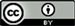1山东科技大学，电子通信与物理学院，山东 青岛

2矿山灾害预防控制省部共建国家重点实验室培育基地，山东 青岛1. 引言

2. 传感器的制作与原理

I = I 1 + I 2 + 2 I 1 I 2 cos [ 2 π ( n c o e f f − n c l , j e f f ) L / λ ] (1)

Δ φ = 2 π ( n c o e f f − n c l , j e f f ) L / λ = 2 π Δ n e f f L / λ (2)

(2)式中： Δ n e f f 表示纤芯模与包层模的折射率差。结合(1)式和(2)式可知，当 Δ φ 为 π 的奇数倍时即 Δ φ = ( 2 k + 1 ) π 时，干涉光强达到最小，此时干涉谱中相应的干涉谷特征波长可表示为：

λ = 2 ( n c o e f f − n c l , j e f f ) L / ( 2 k + 1 ) = 2 Δ n e f f L / ( 2 k + 1 ) (3)

∂ λ j / ∂ n = − ( 2 L / 2 k + 1 ) ⋅ ( ∂ n c l , j e f f / ∂ n ) (4)

3. 实验结果与数据分析

3.1. 低折射率实验3.1.1. 腐蚀前实验

3.1.2 腐蚀后实验

3.2. 高折射率实验3.2.1. 腐蚀前实验

3.2.2. 腐蚀后实验

4个波谷都向短波方向漂移，且漂移量由短波向长波方向依次为6.8 nm、13.633 nm、18.6 nm和18.667 nm。

4. 结论